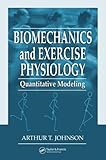Home FAQ Random quotes Contact

# Biomechanics and Exercise Physiology: Quantitative ModelingISBN13: 9781574449068
ISBN: 1574449060
Binding: Hardcover
List Price: \$129.95
Publisher: CRC Press
Published Date:
Edition 2
Pages: 688
Average Goodreads rating: 5.00/5 (1 ratings)

Whether you are a bioengineer designing prosthetics, an aerospace scientist involved in life support, a kinesiologist training athletes, or an occupational physician prescribing an exercise regimen, you need the latest edition of Biomechanics and Exercise Physiology: Quantitative Modeling. Using numerous worked examples to demonstrate what and when to calculate, this book covers more than the fundamentals of exercise physiology and shows you how to calculate responses magnitudes. The second edition improves upon the first edition with inclusion of numerical examples, homework problems, margin notes, and updated material.

The five sections cover the energetics of exercise, biomechanics, circulation, respiration, and thermoregulation. The author explains physiological models, demonstrating the conversion of physiology into quantitative form. Tables of values, diagrams, and figures make this book helpful for estimating magnitudes, determining trends, and illustrating concepts. The book emphasizes quantitative mathematical models if possible and conceptual models when mathematical models are not available.

Covering a broad scope of material, the author emphasizes quantitative description as much as possible. The book demonstrates the vast amount of physiological material that can be quantitatively predicted and how to translate this information into applications.